# Cost Management

## Quiz 7 : Cost Allocation: Departments, Joint Products, and By-ProductsStudy FlashcardsLooking for Management Homework Help?

## Quiz 7 :Cost Allocation: Departments, Joint Products, and By-Products

Question TypeHow does your answer to 7-11 change if the cost in P1 is changed from $100,000 to$120,000 Brief Exercises 7-15 and 7-16 require the following information: Involve departmental cost allocation with two service departments and two production departments. Use the following information for these four exercises: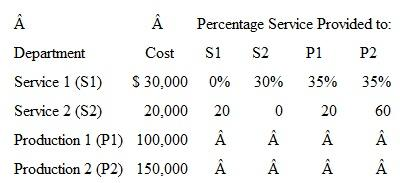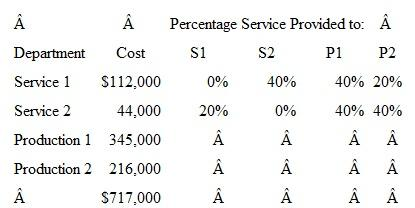Free
Essay

Departmental Cost Allocation:
Departmental cost allocation refers to the allocation of overhead expenses in the department. This approach recognizes that manufacturing operation is divided into production department and support department.
Hence, cost allocation is used to allocate indirect expense between production department and support department.
Direct Method of cost allocation:
This method assumes that services department provides services to the production department only. It is simplest method of allocation because it ignores reciprocal flows.
When the cost of P1 changes from $100,000 to$ 120,000 it will not affect the answer calculated in 7-11 question because 7-11 deals with the cost of service departments allocated to the production department.
For such calculation percentage usage of the production department is service department required and not the cost of production department.
Hence, answer to 7-11 remain unchanged.

Tags
Choose question tagBrief Exercises require the following information: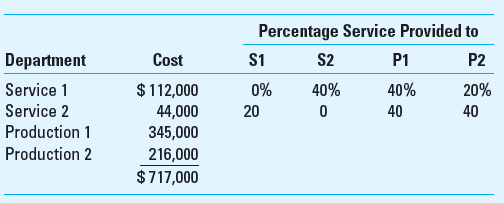What portion of service department 2's costs are allocated to P1 and to P2 under the direct method
Free
Essay

Under the direct method the cost of service departments are allotted only to the production department in the ratio of the percentage for the allocation of production department only.
The allocation of the of S2 would be in the ratio of 1:1 as the percentage is 40% for P1 and P2.The calculation is as follows-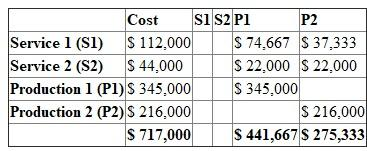Tags
Choose question tagRequire the following information about a joint production process for three products, with a total joint production cost of $100,000. There are no separable processing costs for any of the three products.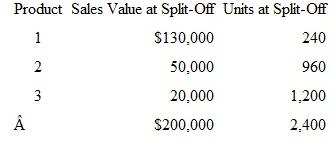Assume the same as in 7-19 above except that product 3 is treated as a joint product. What amount of joint costs would be allocated to the three products using the relative sales value method Free Essay Answer: Answer: Relative Sales Value Method: Relative sales value method is one of the methods of allocating joint cost to the products. It is also known as the Sales value at split off method. In relative sales value method joint cost is allocated to the joint products based on their relative sales value at the split off point. This method is most widely used in allocating joint cost. Calculation of joint cost allocation: Step 1: Calculation of total sales value at split off point:Step 2: Calculation of proportion of sales value for each joint product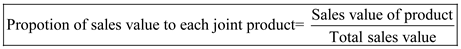Putting the values in the above expression:Step 3: Allocation of joint cost: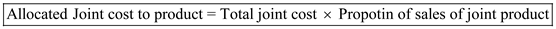Putting the values:Tags Choose question tagWhat are the four objectives in the strategic role of cost allocation Explain each briefly. Essay Answer: Tags Choose question tagRequire the following information about a joint production process for three products, with a total joint production cost of$100,000. There are no separable processing costs for any of the three products.What amount of joint cost is allocated to each of the three products using the relative sales value method
Essay
Tags
Choose question tagWhat does the term reciprocal mean in the context of departmental cost allocation
Essay
Tags
Choose question tagWhat are some of the ethical issues of cost allocation
Essay
Tags
Choose question tagWhat is the total cost in P1 and in P2 after allocation using the direct method
Essay
Tags
Choose question tagBrief Exercises require the following information:What portion of service department 1's costs are allocated to P1 and to P2 under the direct method
Essay
Tags
Choose question tagExplain the difference between joint products and by-products.
Essay
Tags
Choose question tagBrief Exercises require the following information about a joint production process for three products, with a total joint production cost of $100,000. There are no separable processing costs for any of the three products.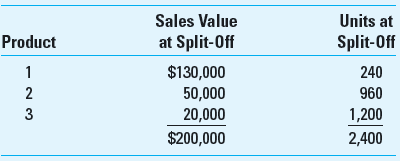What amount of joint cost is allocated to each of the three products using the physical units method Essay Answer: Tags Choose question tagRequire the following information about a joint production process for three products, with a total joint production cost of$100,000. There are no separable processing costs for any of the three products.Assume that the total sales value at the split-off point for product 1 is $50,000 instead of$130,000 and the sales value of product 3 is $2,000 instead of$20,000. Assume also that, because of its rela­tively low sales value, the firm treats product 3 as a by-product and uses the net realizable value method for accounting for joint costs. There are no separable processing costs for product 3. What amount of joint cost would be allocated to the three products
Essay
Tags
Choose question tagInvolve departmental cost allocation with two service departments and two production departments. Use the following information for these four exercises:What is the amount of service department cost allocated to P1 and P2 using the step method with S1 going first
Essay
Tags
Choose question tagWhat are the limitations of joint product cost allocation
Essay
Tags
Choose question tagWhat are the three methods of departmental cost allocation Explain how they differ, which is the most preferred, and why.
Essay
Tags
Choose question tagInvolve departmental cost allocation with two service departments and two production departments. Use the following information for these four exercises:What is the amount of service department cost allocated to P1 and P2 using the direct method
Essay
Tags
Choose question tagWhat are the implementation issues of departmental cost allocation
Essay
Tags
Choose question tagWhat are the three phases of the departmental allocation approach What happens at each phase
Essay
Tags
Choose question tagGive two or three examples of the use of cost allocation in service industries and not-for-profit organizations.
Essay